Review

For questions 1 - 5, use the ordered tree below.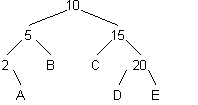1. Which position would hold the number 11?

2. What is the height of the tree?

A. 10 5 2 A B 1 5 C 20 D E
B. 10 5 15 2 B C 20 A D E
C. 2 A 5 B 10 C 15 D 20 E
D. A 2 B 5 C D E 20 15 10
E. A 2 B 5 10 15 C 20 D E

3. From the lists above, which one is an inorder traversal?

4. Which list is a preorder traversal?

5. Which list is a postorder traversal?

Use the trees below to answer questions 6 and 7.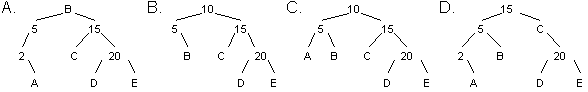6. Which of the trees above would be the resulting tree after the node 2 was deleted from the original tree from problem 1 above?

7. Which of the tree above would be the resulting tree after the node 10 was deleted from the original tree above?

8. If there are 8 internal nodes (not leaves) in a binary tree, at most how many leaves can there be?
A. 1
B. 4
C. 8
D. 9
E. 16

9. Evaluate the binary expression tree.
 A. 23    B. 34    C. 90    D. 120    E. 232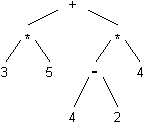10. Which is not true about siblings?
A. They will always have the same number of ancestors.
B. In a binary tree a given node can have two siblings.
C. They will always have the same parent.
D. They will always be on the same level in the tree.

Questions 11 - 13 are base on the following pseudocode for a function P that copies items from an array A containing N distinct items into a binary search tree T and then prints the items. Function P

• Step 1: Initialize binary search tree T to be empty.
• Step 2: For i = 0 to N-1, use the standard algorithm for insertion into a binary search tree to insert item a[i] into T.
• Step 3: Print the items stored in T, using an inorder traversal. Assume that the insert operation used in Step 2 does no balancing of T.

11. Which of the following best characterizes the output produced in Step 3 of function P?
A. The items are printed in the same order in which they appear in array A.
B. The items are printed in sorted order.
C. The items are printed in random order.
D. the items are printed in reverse of the order in which they appear in array A.
E. The value stored in A is printed N times.

12. Which of the following best describes the worst-case running time of function P?
A. O ( 1 )
B. O ( log N )
C. O ( N )
D. D. O ( N log N )
E. E. O ( N2 )

13. Function P must exhibit its worst-case running time when the items are stored in array A in which of the following ways?
• i. Ascending order
• ii. Descending order
• iii. Alternating positive and negative numbers
A. i only
B. ii only
C. iii only
D. i and ii only
E. i, ii, and iii

14. Which of the following lists the steps necessary to add a new node to an ordered binary tree?
A. Use the new function to create the new node, fill the data fields of the new node, set its point fields to `NULL`, and insert the new node at the root.
B. Use the new function to create the new node, fill the data fields of the new node, set its pointer fields to `NULL`, locate the correct location for the node to be added as a leaf, and change the parent's pointer so that it points to the new node.
C. Use the new function to create the new node, fill the data fields of the new node, determine the correct location of the new node and set its pointer field to point to its parent node.
D. Use the new function to create the new node, fill the data fields of the new node, set its right pointer to point to its parent node and its left pointer to `NULL`.

15. The most difficult situation encountered in deleting a node from a binary tree is when:
A. the tree only has one node
B. the node is a leaf
C. the node has a single subtree
D. the node has two subtrees

16. Which of the following program segments could be used to delete a node that is a leaf from a binary tree? Assume that `Current` points to the node being deleted and `Parent` points to the parent of that node.
1. ```if (parent.getRight() == current)    parent.setRight(NULL); else parent.getLeft() = NULL;```
2. ```if (current == current.getRight())    parent.setRight(NULL); else parent.setLeft(NULL);```
3. ```if (! current.getRight() )    parent.setRight(NULL); else parent.setLeft(NULL);```
4. ```if ( parent == current)    parent.setValue(NULL);```

Consider the declaration below.

```int m (int i, int j)
{
if (i >= j)
return i;
else  return j;
}

int f (TreeNode t)
{
if ( t.getLeft() == NULL && t.getRight() == NULL)
return  t.getValue();
if (t.getLeft() == NULL)
return m (t.getValue(), f(t.getRight()));
if (t.getRight() == NULL)
return m(t.getValue(), f( t.getLeft()));
return m ( m ( t.getValue(), f ( t.getLeft())), f (t.getRight()));
}
```

17. If upon entrance to `f`, `t != NULL`, then `f` evaluates to which of the following?

1. The greatest `Value` in the tree
2. The least `Value` in the tree
3. The `Value` at the root of the tree
4. The `Value ` at the last node of the tree that was visited during the execution of `f`
5. The first duplicated `Value `encountered during the execution of `f`

18. In the binary search tree at right, what is the greatest number of key comparisons that will be necessary to determine whether or not a letter is present?
 2 3 4 5 none of the above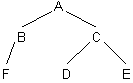19. The difference between an ordered tree and a nonordered tree is ...
1. A nonordered tree can be empty whereas an ordered tree must have at least one node.
2. In a nonordered tree the relative positions of the subtrees are significant whereas in an ordered tree they are not significant.
3. In an ordered tree the relative positions of the subtrees are significant whereas in a nonordered tree they are not significant.
4. An ordered tree has a root node whereas a nonordered tree does not.

Suppose a binary tree is defined as follows.

Consider the following function

``` int D (TreeNode t) {    if ( t.getValue() == NULL)       return 0;    return 1 + Max(Height(t.getLeft()) +       Height(t.getRight()),D(t.getLeft()),D(t.getRight())) } ```

20. Suppose that function `Max` returns the largest of its three integer arguments, and function `Height` returns the height of its tree argument, where height of a tree is defined as follows.

• The height of an empty tree is 0.
• The height of a nonempty tree is the number of nodes on the longest path from the root to a leaf of the tree.

What value is returned when `D` is passed an argument representing the following tree of height 5?
 4 5 6 8 10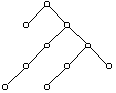Consider the following tree and traversals.

 A B H I C D F G E H I B A F D G C E I H B F G D E C A A B C D E F G H I I H G F E D C B A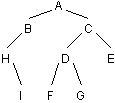21. A inorder traversal of the tree would produce which output?

22. A preorder traversal of the tree would produce which output?

23. A postorder traversal of the tree would produce which output?

24. Each of the following sequences of characters, proceeding from left to right, are to be placed in an initially empty binary search tree. Draw a diagram of the final state of each tree.
• D E M O C R A T S
• R E P U B L I C A N S

Use the following TYPE declaration for questions 25 and 26.

```int Treesum (TreeNode P)
{
int temp = 0;
if ( P.getValue()!=NULL )
temp = Treesum(P.getLeft()) +
Treesum(P.getRight()) + P.getValue();
return temp;
}
```

25. For which trees will the function above work?
1. only empty trees
2. only non-empty trees
3. all trees
4. no trees

```int Treesum (TreeNode P)
{
if ( P.getValue()!=NULL )
{
int temp = 0;
temp = Treesum(P.getLeft()) +
Treesum(P.getRight()) + P.getValue();
}
return temp;
}
```

26. For which trees will the function above work?
1. only empty trees
2. only non-empty trees
3. all trees
4. no trees

Completion

27. Draw the tree representation for the prefix expression: ` a + b * c + d e`

28. Write the postorder transversal of the tree in problem 27.

29. A binary tree of integers is stored in a 4 x 3 matrix with rows corresponding to nodes of the tree. The columns contain the following data:

• column 1: row index of left child
• column 2: info
• column 3: row index of right child

A row index of 0 indicates no child.

Sketch the tree represented by this matrix.

 0 4 0 0 3 3 0 5 0 2 7 1

30. An array implementation of a binary tree could work as follows: The root of the tree is the node at t; the left child of the node t[k] could be a t[2k], and the right child of node t[k] could be at t[2k+1]. What would the corresponding location of the left-most and right-most children of node t[k] in a ternary tree? Quaternary tree? and n-nary tree?

Continue to:  Unit 8 / Prev / Next